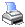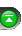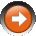﻿ Regression Channel
 NinjaScript > Language Reference > Indicator Methods > Regression ChannelDescription

A Regression Channel is created by drawing parallel lines above and below the Linear Regression line.

Parallel and equidistant lines are drawn n standard deviations (width parameter) above and below a Linear Regression trendline. The distance between the channel lines and the regression line is the greatest distance that any one closing price is from the regression line. Regression Channels contain price movement, the top channel line provides resistance and the bottom channel line provides support. A reversal in trend may be indicated when prices remain outside the channel for a longer period of time.

A Linear Regression trendline shows where equilibrium exists but Linear Regression Channels show the range prices can be expected to deviate from a trendline.

Syntax

 RegressionChannel(int period, int width) RegressionChannel(IDataSeries input, int period, int width)   Returns default midline value RegressionChannel(int period, int width)[int barsAgo] RegressionChannel(IDataSeries input, int period, int width)[int barsAgo]   Returns upper channel value RegressionChannel(int period, int width).Upper[int barsAgo] RegressionChannel(IDataSeries input, int period, int width).Upper[int barsAgo]   Returns lower channel value RegressionChannel(int period, int width).Lower[int barsAgo] RegressionChannel(IDataSeries input, int period, int width).Lower[int barsAgo]

Return Value

double; Accessing this method via an index value [int barsAgo] returns the indicator value of the referenced bar.

Parameters

 input Indicator source data (?) period Number of bars used in the calculation width Number of std deviations to calculate the channel lines

Tips

You should not access historical values of this indicator since the values can change from bar to bar. The values from n bars ago does not reflect what the values of the current bar really are. It is suggested that you only access the current bar value for this indicator.

Examples

 // Prints the current value of a 20 period channel using default price type double value = RegressionChannel(20, 2).Upper; Print("The current upper channel value is " + value.ToString());

Source Code

You can view this indicator method source code by selecting the menu Tools > Edit NinjaScript > Indicator within the NinjaTrader Control Center window.